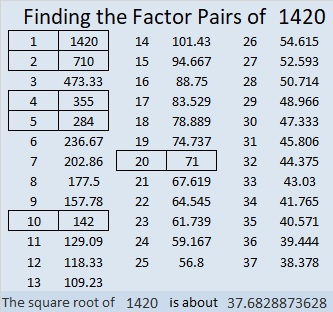# 1420 Eight-legged Creature

When you look at today’s puzzle, you might see a snowflake or perhaps an eight-legged creature. I hope you will also see lots of common factors that will help you solve this quick puzzle.Print the puzzles or type the solution in this excel file: 12 Factors 1419-1429

Here are some facts about the puzzle number, 1420:

• 1420 is a composite number.
• Prime factorization: 1420 = 2 × 2 × 5 × 71, which can be written 1420 = 2² × 5 × 71.
• 1420 has at least one exponent greater than 1 in its prime factorization so √1420 can be simplified. Taking the factor pair from the factor pair table below with the largest square number factor, we get √1420 = (√4)(√355) = 2√355.
• The exponents in the prime factorization are 2, 1, and 1. Adding one to each exponent and multiplying we get (2 + 1)(1 + 1)(1 + 1) = 3 × 2 × 2 = 12. Therefore 1420 has exactly 12 factors.
• The factors of 1420 are outlined with their factor pair partners in the graphic below.1420 is the hypotenuse of a Pythagorean triple:
852-1136-1420 which is (3-4-5) times 284.